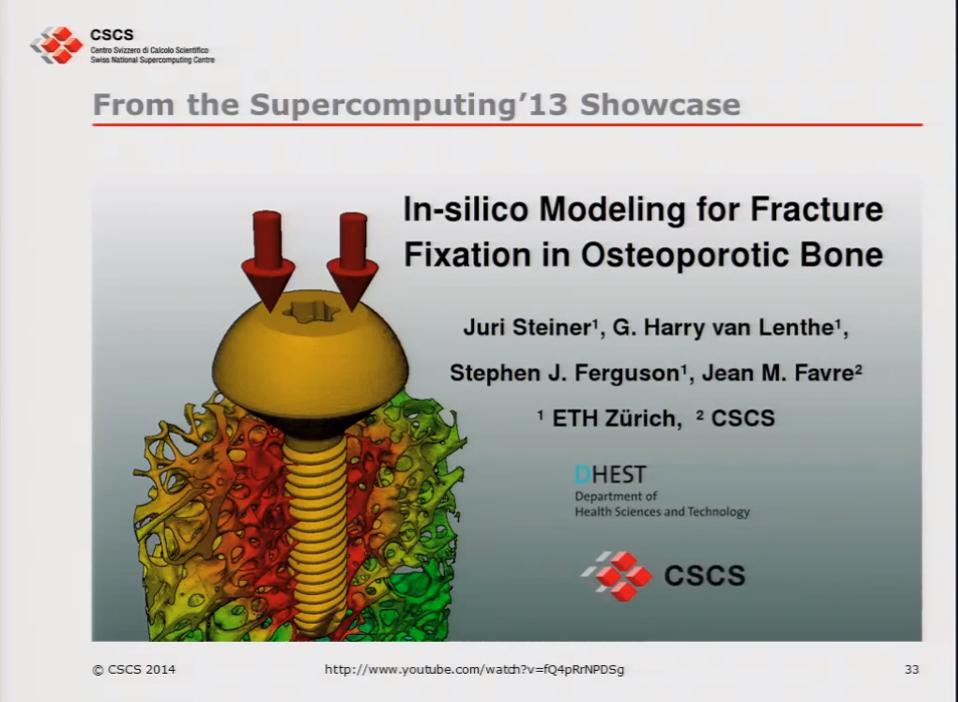# Slidecasts (1/2): Visualization and Graphics Tutorial at CSCS

CSCS organized in January a two day course on visualization and graphics. Speaker was Jean Favre (CSCS).

We publish the slidecasts of the course starting with day 1.

• Introduction, objectives
• Matplotlib, a python 2D data plotting library
• VTK Charts and Infovis: Introduction by example
• The Visualization Pipeline. Introduction
• Data formatting for 3D Visualization
• ParaView, an open-source turn-key 3D data visualizer
• ParaView-specific Features
• Parallel and client-server usage from the outside to CSCS
• Interactive sessions vs. batch-oriented scripting

Visualization and Graphics Tutorial 1/7: matplotlib, Visualization Pipeline and InfoVis

Visualization and Graphics Tutorial 2/7: Visualization Pipeline (cont’d)

Visualization and Graphics Tutorial 3/7: Data formats for Visualization

Visualization and Graphics Tutorial 4/7: ParaView introduction from SNL tutorial